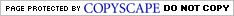# Craps Fibonacci System

by Larry Edell

This Learn to Play Craps lesson reveals the Craps Fibonacci System
This is a somewhat advanced system but once you get used to it, you'll see that it almost always wins if you stick to the rules and don't make any other bets.

Fibonacci is a mathematical term and means that you have a number series where the total of each two consecutive numbers is larger than all of the preceding numbers.
For example, a simple Fibonacci series would be 1, 1, 2, 3, 5, 8, 13. As you can see, 13 + 8 (21) is more than 1 + 1 + 2 + 3 + 5 (12).  Also 5 + 8 (13) is more than 1 + 1 + 2 + 3 (7).

Another attribute to this series is that the next number would be the sum of the two previous numbers.
For example, 13 is the sum of 8 +5. Also, the next number after 13 would be 13 + 8 or 21.

The Craps Fibonacci System or mathematical series can be very profitable to a crapshooter. You can bet flat bets on the pass, don't pass, come or don't come.
If you win any two consecutive bets, no matter where you are on the progression you will make money. And, if you win any two out three bets, you will also have a profit if you stick to the progression.

The progression you must bet is, of course, the Craps Fibonacci system series.
You will bet multiples of 1, 1, 2, 3, 5, 8, 13, 21, 34, 55, 89, 144, etc.
With the exception of the first two bets, your new wager is always the sum of your prior two bets, so you don't have to memorize anything.

Let's look at some examples of the Craps Fibonacci System.
For all of these we will assume \$1 unit bets.
You start betting, first \$1 on the pass line, lose, and bet another \$1. Now once the first two bets are established, the next bet is always the total of the 2 previous bets, so you bet \$2, which you lose also.
The next bet is \$3 (\$2 + \$1) which you lose. 3 +2 is 5 so your fifth bet is \$5, easy to remember, but you lose that one also.
Now your sixth bet is \$5 plus \$3 or \$8 which you win. Your seventh bet is \$8 + \$5 or \$13 which you win also.

Now let's see what happened.
You lost the first five bets - \$1 + \$1 + \$2 + \$3 + \$5 or a total of \$12. You win the next two bets for \$8 and \$13, so you've won \$21 and lost \$12 so you have a \$9 profit.
Let's say you lose the first ELEVEN bets so you lose \$1 + \$1 + \$2 + \$3 +\$5 + \$8 + \$13 + \$21 + \$34 + \$55 + \$89 or a total of \$232.
If you win only the next two bets at \$144 and \$233, you would win \$377 and be ahead by \$144.

You will also profit if you win any two out of three bets.
If you won the eleventh bet of \$89, lost the twelfth bet of \$144 but won the thirteenth bet of \$233, you would still be ahead by \$178!

Your goal in this system is to make a profit. Once you win any two out of three bets, you pocket the profit and start again at bet # 1.
You should quit when you win 50% of your buy in, so if you buy in for \$500 you should stop when you win \$250.

Craps Fibonacci System is followed by Field vs. Inside Betting
OR

Gambling Teachers home## Tips, Terms & Wins

Craps Numbers: 9 or “Nina from Pasadena” is the Center Field  and can be made four ways: 6:3, 3:6, 5:4, and 4:5. Nine is often Placed in conjunction with the 5 and, when coupled with the 6 and 8, is one of the “inside”; when coupled with the 4 or 10 is considered one of the “outside.”